# Eigenvalue and Eigenvector in Linear Algebra: Tutoring Solution Chapter Exam

Exam Instructions:

Choose your answers to the questions and click 'Next' to see the next set of questions. You can skip questions if you would like and come back to them later with the yellow "Go To First Skipped Question" button. When you have completed the practice exam, a green submit button will appear. Click it to see your results. Good luck!

### Page 1

#### Question 1 1. In order to find a matrix's eigenvectors, we solve the following equation. Which of this equation's variables represents the eigenvectors?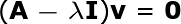#### Question 3 3. Find the eigenvectors associated with the eigenvalue of 4 for the following matrix.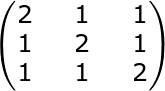#### Question 4 4. Find the eigenvectors associated with the eigenvalue of 4 for the following matrix.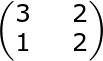#### Question 5 5. Find the eigenvectors associated with the eigenvalue of 0 for the following matrix.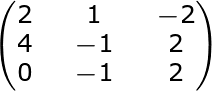### Page 3

#### Question 12 12. One of the eigenvalues of the following matrix is -2. Find the corresponding eigenvector where we put 1 in for the first parameter of the vector.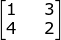#### Question 13 13. For any real number x, the following is an eigenvector of a matrix A. Find its unit eigenvector.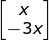#### Question 14 14. Find the eigenvalues of the following matrix.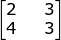### Page 4

#### Question 16 16. Find all the eigenvalues for the following 3x3 matrix (A).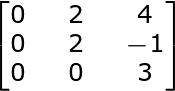#### Question 19 19. Find all the eigenvalues for the following 2x2 matrix (A).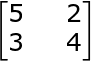#### Question 20 20. Find all the eigenvalues of the following 2x2 matrix (A).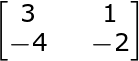### Page 5

#### Question 21 21. The matrix, A, is a _____.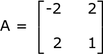#### Question 22 22. To diagonalize a symmetric matrix, A, we calculate Pt AP. The result is the matrix, D, where D is the diagonal matrix shown. The eigenvalues of A are _____.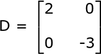#### Question 23 23. For the eigenvector, v, the normalized eigenvector, u, is _____.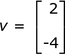#### Question 24 24. To diagonalize a matrix, A, we calculate Pt AP. For the normalized eigenvectors, u1 and u2, the matrix, P, is given by _____.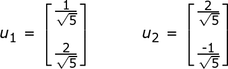#### Question 25 25. If the eigenvector of a matrix is the vector, v1, shown below, then the length of v1 is _____.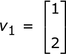#### Eigenvalue and Eigenvector in Linear Algebra: Tutoring Solution Chapter Exam Instructions

Choose your answers to the questions and click 'Next' to see the next set of questions. You can skip questions if you would like and come back to them later with the yellow "Go To First Skipped Question" button. When you have completed the practice exam, a green submit button will appear. Click it to see your results. Good luck!

Support